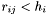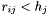# ngb.c File Reference

neighbour search by means of the tree More...

`#include <stdio.h>`
`#include <stdlib.h>`
`#include <string.h>`
`#include <math.h>`
`#include <time.h>`
`#include <mpi.h>`
`#include "allvars.h"`
`#include "proto.h"`

Go to the source code of this file.

## Defines

#define boxSize_X   boxSize
#define boxHalf_X   boxHalf
#define boxSize_Y   boxSize
#define boxHalf_Y   boxHalf
#define boxSize_Z   boxSize
#define boxHalf_Z   boxHalf
#define NGB_PERIODIC_X(x)   (xtmp=(x),(xtmp>boxHalf_X)?(xtmp-boxSize_X):((xtmp<-boxHalf_X)?(xtmp+boxSize_X):xtmp))
#define NGB_PERIODIC_Y(x)   (xtmp=(x),(xtmp>boxHalf_Y)?(xtmp-boxSize_Y):((xtmp<-boxHalf_Y)?(xtmp+boxSize_Y):xtmp))
#define NGB_PERIODIC_Z(x)   (xtmp=(x),(xtmp>boxHalf_Z)?(xtmp-boxSize_Z):((xtmp<-boxHalf_Z)?(xtmp+boxSize_Z):xtmp))

## Functions

int ngb_treefind_pairs (float searchcenter, float hsml, int *startnode)
int ngb_treefind_variable (float searchcenter, float hsml, int *startnode)
int ngb_clear_buf (float searchcenter, float hsml, int numngb)
void ngb_treeallocate (int npart)
void ngb_treefree (void)
void ngb_treebuild (void)

## Detailed Description

neighbour search by means of the tree

This file contains routines for neighbour finding. We use the gravity-tree and a range-searching technique to find neighbours.

Definition in file ngb.c.

## Define Documentation

 #define boxHalf_X   boxHalf
 Definition at line 26 of file ngb.c.

 #define boxHalf_Y   boxHalf
 Definition at line 32 of file ngb.c.

 #define boxHalf_Z   boxHalf
 Definition at line 38 of file ngb.c.

 #define boxSize_X   boxSize
 Definition at line 25 of file ngb.c.

 #define boxSize_Y   boxSize
 Definition at line 31 of file ngb.c.

 #define boxSize_Z   boxSize
 Definition at line 37 of file ngb.c.

 #define NGB_PERIODIC_X ( x ) (xtmp=(x),(xtmp>boxHalf_X)?(xtmp-boxSize_X):((xtmp<-boxHalf_X)?(xtmp+boxSize_X):xtmp))
 these macros maps a coordinate difference to the nearest periodic image Definition at line 47 of file ngb.c. Referenced by ngb_clear_buf(), ngb_treefind_pairs(), and ngb_treefind_variable().

 #define NGB_PERIODIC_Y ( x ) (xtmp=(x),(xtmp>boxHalf_Y)?(xtmp-boxSize_Y):((xtmp<-boxHalf_Y)?(xtmp+boxSize_Y):xtmp))
 Definition at line 48 of file ngb.c. Referenced by ngb_clear_buf(), ngb_treefind_pairs(), and ngb_treefind_variable().

 #define NGB_PERIODIC_Z ( x ) (xtmp=(x),(xtmp>boxHalf_Z)?(xtmp-boxSize_Z):((xtmp<-boxHalf_Z)?(xtmp+boxSize_Z):xtmp))
 Definition at line 49 of file ngb.c. Referenced by ngb_clear_buf(), ngb_treefind_pairs(), and ngb_treefind_variable().

## Function Documentation

 int ngb_clear_buf ( float searchcenter, float hsml, int numngb )
 The buffer for the neighbour list has a finite length MAX_NGB. For a large search region, this buffer can get full, in which case this routine can be called to eliminate some of the superfluous particles in the "corners" of the search box - only the ones in the inscribed sphere need to be kept. Definition at line 320 of file ngb.c. References FLOAT, NGB_PERIODIC_X, NGB_PERIODIC_Y, NGB_PERIODIC_Z, Ngblist, P, and particle_data::Pos. Referenced by ngb_treefind_variable().

 void ngb_treeallocate ( int npart )
 Allocates memory for the neighbour list buffer. Definition at line 358 of file ngb.c. Referenced by init(), and restart().

 void ngb_treebuild ( void )
 This function constructs the neighbour tree. To this end, we actually need to construct the gravitational tree, because we use it now for the neighbour search. Definition at line 403 of file ngb.c. References force_treebuild(), N_gas, and ThisTask. Referenced by init().

 int ngb_treefind_pairs ( float searchcenter, float hsml, int * startnode )
 This routine finds all neighbours `j' that can interact with the particle `i' in the communication buffer. Note that an interaction can take place ifOR if. In the range-search this is taken into account, i.e. it is guaranteed that all particles are found that fulfil this condition, including the (more difficult) second part of it. For this purpose, each node knows the maximum h occuring among the particles it represents. < defines maximum length of neighbour list Definition at line 64 of file ngb.c. Referenced by hydro_evaluate().

 int ngb_treefind_variable ( float searchcenter, float hsml, int * startnode )
 This function returns neighbours with distance <= hsml and returns them in Ngblist. Actually, particles in a box of half side length hsml are returned, i.e. the reduction to a sphere still needs to be done in the calling routine. < defines maximum length of neighbour list < defines maximum length of neighbour list Definition at line 194 of file ngb.c. Referenced by density_evaluate().

 void ngb_treefree ( void )
 free memory allocated for neighbour list buffer. Definition at line 394 of file ngb.c. References Ngblist.

Generated on Sun May 22 17:33:30 2005 for GADGET-2 by1.3.9.1